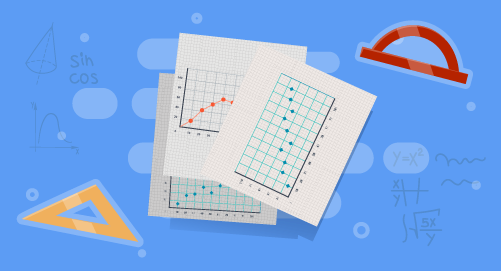#### Need Help?

Get in touch with usSAT/ACT

# Statistics

Maths

Construct and interpret scatter plots for bivariate measurement data. A linear association that fits a straight line, and assess the model fit by judging the closeness of the data points to the line. Use the equation of a linear model to solve problem in the context of bivariate measurement data. Construct and interpret a two-way table summarizing data on two categorical variables. Use relative frequencies to describe possible association between the two variable.

##### Course Duration
•2 Weeks5 Sessions
•45 mins per session
##### Tutor## What you'll learn

• 1

Construct a scatter plot and use it to understand the relationship between paired data.

• 2

Use a line to represent the relationship between paired data.

• 3

Make prediction by using the equation of a line that closely fits a set of data.

• 4

Display and interpret relationships between paired categorical data.

• 5

Find the relative frequencies of two-way tables and interpret them.

## Skills you'll learnInfering graphs